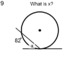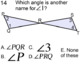# 25 Socrative High School Math Assignments (Part 1) for SMART NotebookSubject
Resource Type
File Type
Zip (42 MB|50 pages)
\$4.00
Also included in:
1. Included in this zip folder are 75 high school math assignments on SMART Notebook files. These assignments can be completed at socrative.com or be printed. Each is on 1 page for easy printing. The 1st assignment is free in the preview section. A brief description and title of each:Part 1(1) 2 Way Fr
\$12.00
\$10.00
Save \$2.00
Product Description
Included in this zip folder are 25 high school math assignments in SMART Notebook files. These assignments can be completed at socrative.com, used with SMART Response or be printed. Each is on 1 or 2 pages for easy printing. The 1st assignment is free in the preview section. A brief description and title of each:

(1) 2 Way Frequency Tables Assignment #1B
Reading a 2 way table and probability

(2) 2 Way Frequency Tables Assignment #1C
Reading a 2 way table and probability

(3) 2 Way Frequency Tables Assignment #2
Reading a 2 way table and probability

(4) Analyze and Identify a Parent Function Assignment #4
Identify properties of a function such as end behavior, asymptotes, intervals of increase and decrease, domain range ans symmetry.

(5) Analyze and Identify a Parent Function Assignment #5
Like #4

(6) Analyze and Identify a Parent Function Assignment #6
Like #4

(7) Angles in Geometry Assignment #1
Naming an angle

(8) Angles in Geometry Assignment #1A
Naming an angle

(9) Angles in Geometry Assignment #1B

(10) Angles in Geometry Assignment #3
Supplementary and complementary angles, adjacent angles and linear pair

(11) Characteristics of Parabolas Assignment #2
Transformations in function equations

(12) Characteristics of Parabolas Assignment #3
Shifts and reflections

(13) Characteristics of Parabolas Assignment #5
Multiple transformations

(14) Characteristics of Parabolas Assignment #6
Stretches and shrinks of parabolas

(15) Characteristics of Parabolas Assignment #7
Axis of Symmetry

(16) Characteristics of Parabolas Assignment #8
Interval of increase and decrease

(17) Characteristics of Parabolas Assignment #12
Transforming f(x) to g(x)

(18) Characteristics of Parabolas Assignment #13
Like the previous assignment

(19) Circle Arcs and Angles Assignment A
Definitions like secant, tangent, chord, minor and major arc

(20) Circle Arcs and Angles Assignment AA
Like the previous

(21) Circle Arcs and Angles Assignment AAA
How angles intercept arcs

(22) Circle Arcs and Angles Assignment E
Find central, inscribed, interior and exterior angles given arcs

(23) Circle Arcs and Angles Assignment F
Find arcs given angles

(24) Circle Arcs and Angles Assignment G
Like the previous

(25) Circle Arcs and Angles Assignment H
Like the previous

Thank you for looking

Total Pages
50 pages